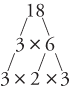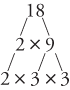## Factors, Primes, Composites, and Factor Trees

You should become familiar with the definitions of certain types of numbers and how they can be found.

#### Factors

Numbers that are multiplied together to get a product are called factors.

##### Example 1

What are the factors of 18?

factor × factor = 18

1 × 18 = 18

2 × 9 = 18

3 × 6 = 18

So, the factors of 18 are 1, 2, 3, 6, 9, and 18. These numbers are also called the divisors of 18. Factors of a number are also called divisors of that same number.

#### Prime numbers

prime number is a natural number, greater than 1, that can be divided by only itself and 1. Another definition: A prime number is a positive integer that has exactly two different factors: itself and 1.

##### Example 2

Is 19 a prime number?

Yes. The only factors of 19 are 1 and 19, so 19 is a prime number. That is, 19 is divisible by only 1 and 19, so it is prime.

##### Example 3

Is 27 a prime number?

No. 27 is divisible by other numbers (3 and 9), so it is not prime. The factors of 27 are 1, 3, 9, and 27, so it is not prime.

The only even prime number is 2; thereafter, any even number may be divided by 2. The numbers 0 and 1 are not prime numbers. The prime numbers less than 50 are 2, 3, 5, 7, 11, 13, 17, 19, 23, 29, 31, 37, 41, 43, and 47.

#### Composite numbers

composite number is a natural number divisible by more than just 1 and itself. Another definition: A composite number is a positive integer that has more than two different factors. The numbers 4, 6, 8, 9, 10, 12, 14, 15, 16, 18, … are composite numbers because they are “composed” of other numbers. The numbers 0 and 1 are not composite numbers. (They are neither prime nor composite.)

##### Example 4

Is 25 a composite number?

Yes. 25 is divisible by 5, so it is composite. The factors of 25 are 1, 5, and 25.

#### Factor trees

Every composite number can be expressed as a product of prime factors. You can find prime factors by using a factor tree. A factor tree looks like this.You can also make the tree as shown in the next tree.In either case, no matter how 18 is factored, the product of the primes is the same, even though the order may be different.

##### Example 5

Use a factor tree to express 60 as a product of prime factors.So the prime factorization of 60 is 2 × 2 × 3 × 5, which can be written as 2 2 × 3 × 5. The actual prime factors of 60 are 2, 3, and 5.